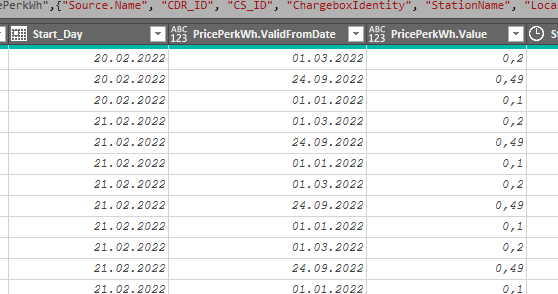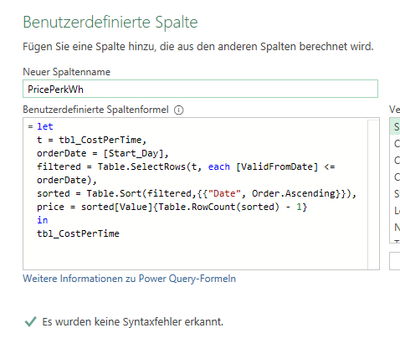cancel
Showing results for
Did you mean:Regular Visitor

## Work with dates

Hello,
I am importing charging data from a charging station for electric cars. To be able to calculate the cost of the charged energy, the date when the charging was started is important.

The cost per kWh can vary over the year.

I have an excel table (Table1) with 2 columns. in the first column there is a date. in the second column there is an amount of money.

Every time the price for a kWh changes, a new row is inserted in this table with the date of the price change and the new price:

 ValidFrom Price 20.02.2022 0,1 01.08.2022 0,5

With PowerQuery I now want to compare the date of a start of a charging process with the data in Table1.

For a charge start on 20.03.2022 the price of 20.02.2022 should be used for the calculation. For a charge start on 02.08.2022, the price from 01.08.2022 should be used for the calculation.

How can I implement this in PowerQuery with a user-defined column?

Can anyone help me here? So far I do not get this done with my knowledge. In a separate query I have tried it with "<=". But here PowerQuery whines that this comparison operators is not possible when using Date/Time.

Best regards
Ralph

1 ACCEPTED SOLUTIONRegular Visitor

Hi Alexandre,

I have written a query with the code (see below). I call this query in a custom column. It always finds the correct price!

``````(FieldInput as datetime) =>
let
qSource = Excel.CurrentWorkbook(){[Name="tbl_CostPerTime"]}[Content],
qRow = Table.SelectRows(qSource, each ([ValidFromDate]<=FieldInput)),
qContent=
Table.Sort(qRow,{{"ValidFromDate", Order.Ascending}})[Value]{Table.RowCount(Table.Sort(qRow,{{"ValidFromDate", Order.Descending}})) - 1}
in
qContent``````

4 REPLIES 4Helper II

Hello @RalphHoffmann , here is what I suggest you to do to achieve your goal:I have 2 tables in my Excel sheet, both imported and typed in PowerQuery.

In the 'Orders' table I aded a custom column to calculate the correct price to use. Here is the formula explained:

let
t = Prices, //we store the 'Prices' table in a variable
orderDate = [Order date], //we store the row order date in a variable
filtered = Table.SelectRows(t, each [Date] <= orderDate), //we filter the 't' table to remove all prices after the order date
sorted = Table.Sort(filtered,{{"Date", Order.Ascending}}), //we sort the 'filtered' table from the oldest date to the newest
price = sorted[Price]{Table.RowCount(sorted) - 1} //the price we want is on the last row of the 'sorted' table: it's the latest price date before the order date
in
price

This could be simplified (and optimized) in these 2 ways:

remove the t = Prices step (I did this because my screen isn't very large, and I didn't wanted to have a single instruction on 2 rows),

set the sorting step directly in the Prices table.

Hope this helps.Regular Visitor

Hi Alexandre,

thank you for your help. Your suggestion does not quite work. As you can see in the screenshots, the date from which a price is valid is entered in the stored order, but not matching the start day of the loading process.

However, the prices must be assigned as follows:

- Charge start 20.02.2022, price from 01.01.2022
- Charge start 07.03.2022, price from 01.03.2022
- Charge start 12.09.2022, price from 01.09.2022

Result:ResultTable with Prices:User defined ColumnUserdefined column

Is it possible that the runtime must be informed in line 4 from which table the column [ValidFromDate] comes?Regular Visitor

Hi Alexandre,

I have written a query with the code (see below). I call this query in a custom column. It always finds the correct price!

``````(FieldInput as datetime) =>
let
qSource = Excel.CurrentWorkbook(){[Name="tbl_CostPerTime"]}[Content],
qRow = Table.SelectRows(qSource, each ([ValidFromDate]<=FieldInput)),
qContent=
Table.Sort(qRow,{{"ValidFromDate", Order.Ascending}})[Value]{Table.RowCount(Table.Sort(qRow,{{"ValidFromDate", Order.Descending}})) - 1}
in
qContent``````Helper II

Hello @RalphHoffmann , you miss some adaptations to the function code:Try to replace (row 6)

sorted = Table.Sort(filtered,{{"Date", Order.Ascending}})

by

sorted = Table.Sort(filtered,{{"ValidFromDate", Order.Ascending}})

And to replace (rows 8 & 9)

in tbl_CostPerTime

by

in price